Courses

# Stress Concentration (Part - 2) Mechanical Engineering Notes | EduRev

## : Stress Concentration (Part - 2) Mechanical Engineering Notes | EduRev

The document Stress Concentration (Part - 2) Mechanical Engineering Notes | EduRev is a part of the Course Machine Design.

Theoretical basis of stress concentration

Consider a plate with a hole acted upon by a stressσ . St. Verant’s principle states that if a system of forces is replaced by another statically equivalent system of forces then the stresses and displacements at points remote from the region concerned are unaffected. In figure-3.2.3.1 ‘a’ is the radius of the hole and at r=b, b>>a the stresses are not affected by the presence of the hole.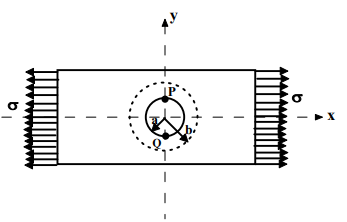3.2.3.1F- A plate with a central hole subjected to a uni-axial stress

Here, σx = σy  , σ = 0, τxy = 0 For plane stress conditions: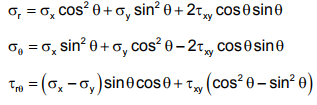This reduces to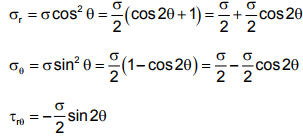such that 1st component in σr and σθ is constant and the second component varies with θ . Similar argument holds for rτθ if we write rτθ =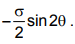The stress distribution within the ring with inner radius ri a = and outer radius or b = due to 1st component can be analyzed using the solutions of thick cylinders and the effect due to the 2nd component can be analyzed following the Stress-function approach. Using a stress function of the form φ = θ R (r) cos2θ the stress distribution due to the 2nd component can be found and it was noted that the dominant stress is the Hoop Stress, given by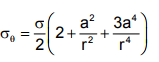This is maximum at θ= ±π 2 and the maximum value of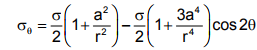Therefore at points P and Q where r =a  σθ is maximum and is given by σθ = 3σ i.e. stress concentration factor is 3.

Offer running on EduRev: Apply code STAYHOME200 to get INR 200 off on our premium plan EduRev Infinity!

## Machine Design

57 videos|71 docs|102 tests